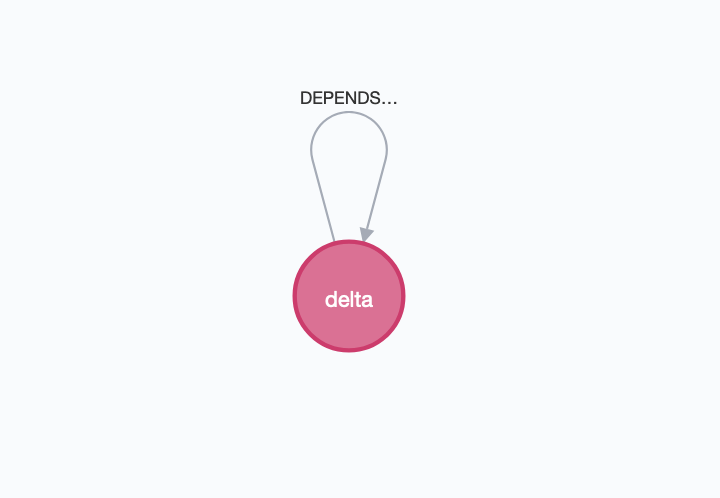# apoc.nodes.cycles

Procedure APOC Core

CALL apoc.nodes.cycles([nodes], \$config) - Detect all path cycles from node list

## Signature

``apoc.nodes.cycles(nodes :: LIST? OF NODE?, config = {} :: MAP?) :: (path :: PATH?)``

## Input parameters

Name Type Default

nodes

LIST? OF NODE?

null

config

MAP?

{}

## Config parameters

Table 1. Config parameters
name type default description

maxDepth

long

Integer.MAX_VALUE

The max number of hops (relationships) to search for cycles.

relTypes

List<String>

[]

The relationship types to detect cycles. By default, all types will be considered.

Name Type

path

PATH?

## Usage Examples

The examples in this section are based on the following sample graph:

``````CREATE (m1:Start {bar: 'alpha'}) with m1 CREATE (m1)-[:DEPENDS_ON {id: 0}]->(m2:Module {bar: 'one'})-[:DEPENDS_ON {id: 1}]->(m3:Module {bar: 'two'})-[:DEPENDS_ON {id: 2}]->(m1)  WITH m1, m2, m3 CREATE (m1)-[:DEPENDS_ON {id: 3}]->(m2), (m2)-[:ANOTHER {id: 4}]->(m3), (m2)-[:DEPENDS_ON {id: 5}]->(m3) CREATE (m1)-[:DEPENDS_ON {id: 6}]->(:Module {bar: 'seven'})-[:DEPENDS_ON {id: 7}]->(:Module {bar: 'eight'})-[:DEPENDS_ON {id: 8}]->(m1);
CREATE (m1:Start {bar: 'beta'}) with m1 CREATE (m1)-[:MY_REL {id: 9}]->(m2:Module {bar: 'three'})-[:MY_REL  {id: 10}]->(m3:Module {bar: 'four'})-[:MY_REL {id: 11}]->(m1);
CREATE (m1:Start {bar: 'gamma'}) with m1 CREATE (m1)-[:DEPENDS_ON {id: 12}]->(m2:Module {bar: 'five'})-[:DEPENDS_ON {id: 13}]->(m3:Module {bar: 'six'});
CREATE (m1:Start {bar: 'delta'}) with m1 CREATE (m1)-[:DEPENDS_ON {id: 20}]->(m1);
CREATE (m1:Start {bar: 'epsilon'}) with m1 CREATE (m1)-[:DEPTH_ONE {id: 30}]->(:Module {bar: 'seven'})-[:DEPTH_ONE {id: 31}]->(m1);``````

The data set above consists in a node `alpha` with 2 cycles, a node `beta` with 1 cycles, a `gamma` node without cycles, a node `delta` with 1 cycle (with only 1 intermediate node), and a `epsilon` node with a self-relationship: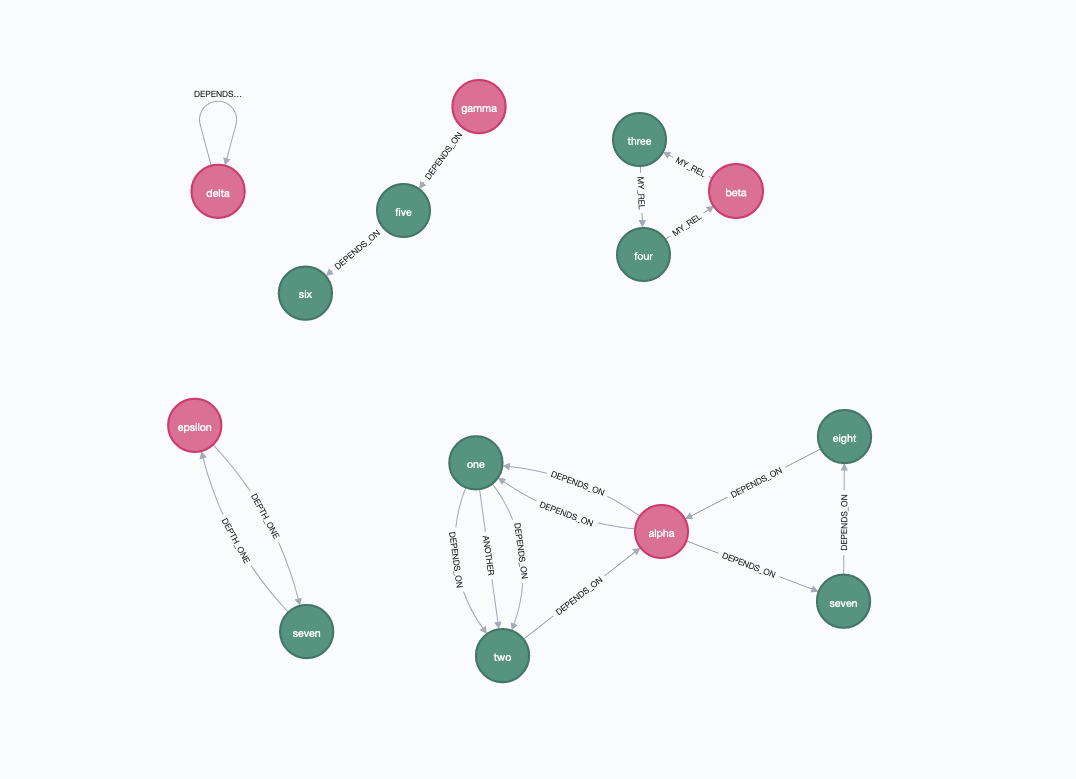We can execute the following query which looks for all the cycles starting from the `Start` nodes:

``MATCH (m1:Start) WITH collect(m1) as nodes CALL apoc.nodes.cycles(nodes) YIELD path RETURN path``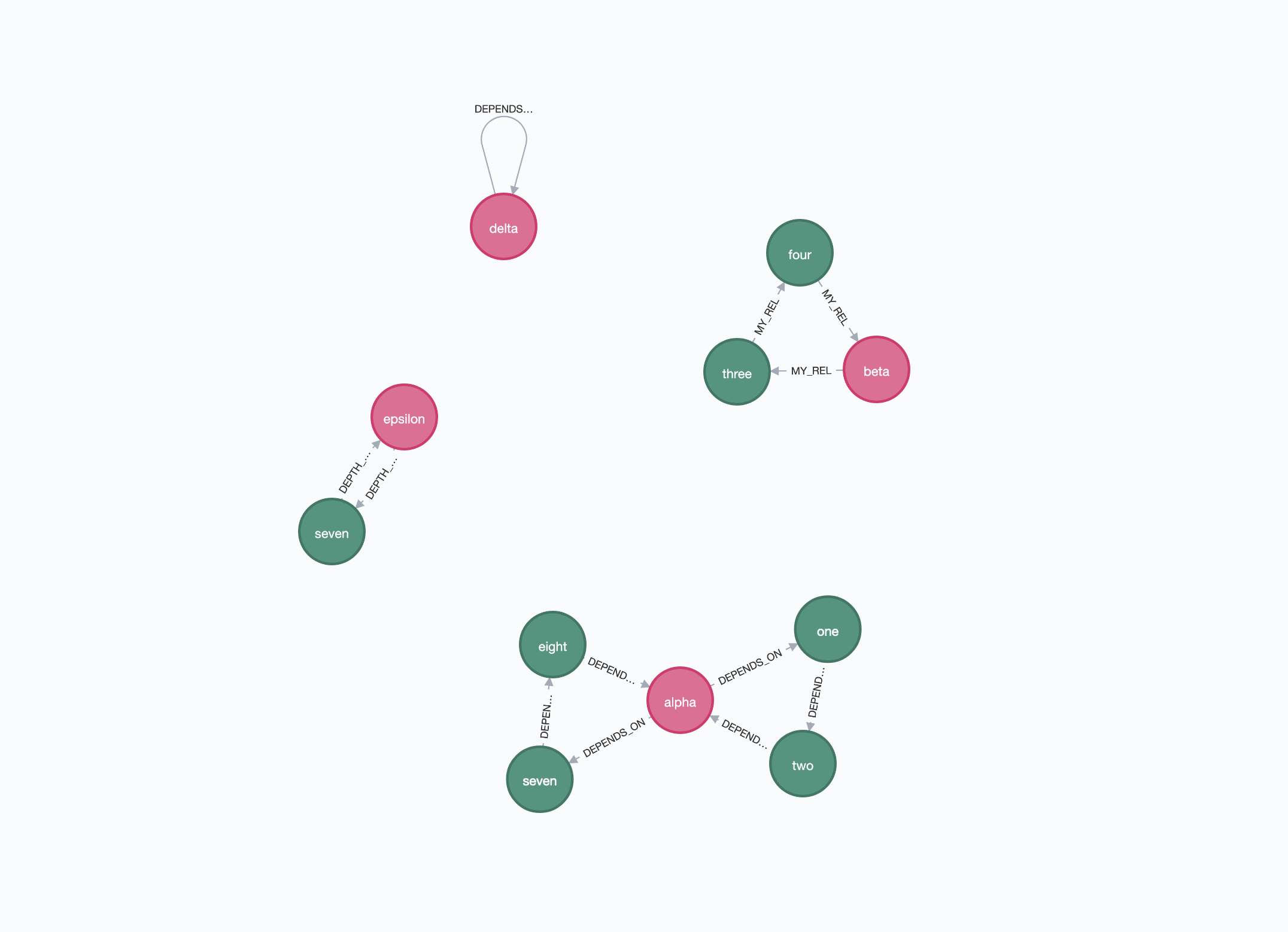Note that, in case of nodes with double-rels (in this example, between `(:Start {bar: 'alpha'})` and `(:Module {bar: 'one'})` and between `(:Module {bar: 'one'})` and `(:Module {bar: 'two'})`), only the first cycle found will be considered.

We can also specify a list of relationship types to detect cycles. For example:

``MATCH (m1:Start) WITH collect(m1) as nodes CALL apoc.nodes.cycles(nodes, {relTypes: ["DEPENDS_ON", "MY_REL", "NOT_EXISTENT"]}) YIELD path RETURN path``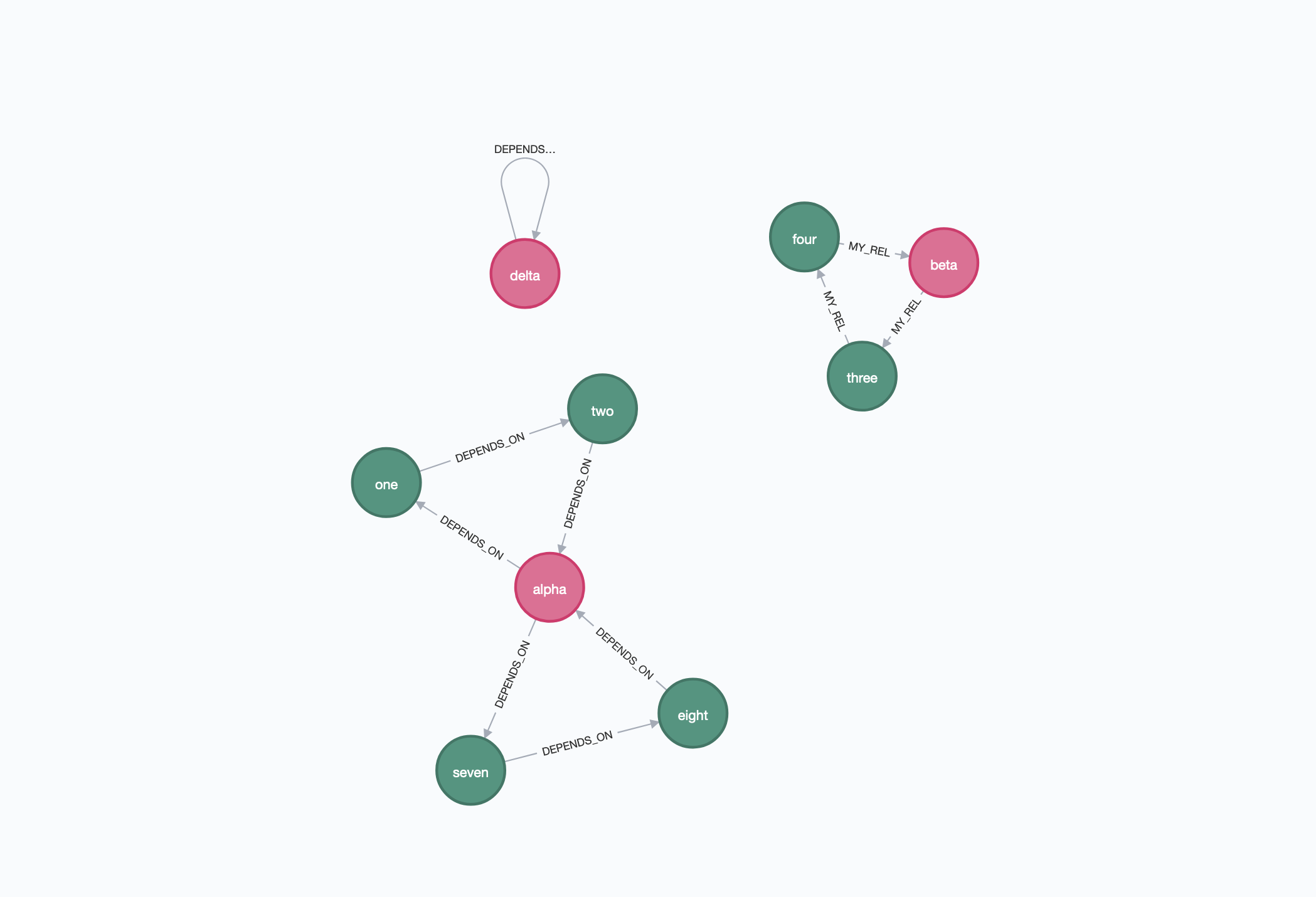Furthermore, we can specify a `maxDepth` to consider cycles with only `n` intermediate nodes. For example:

``MATCH (m1:Start) WITH collect(m1) as nodes CALL apoc.nodes.cycles(nodes, {maxDepth: 1}) YIELD path RETURN path``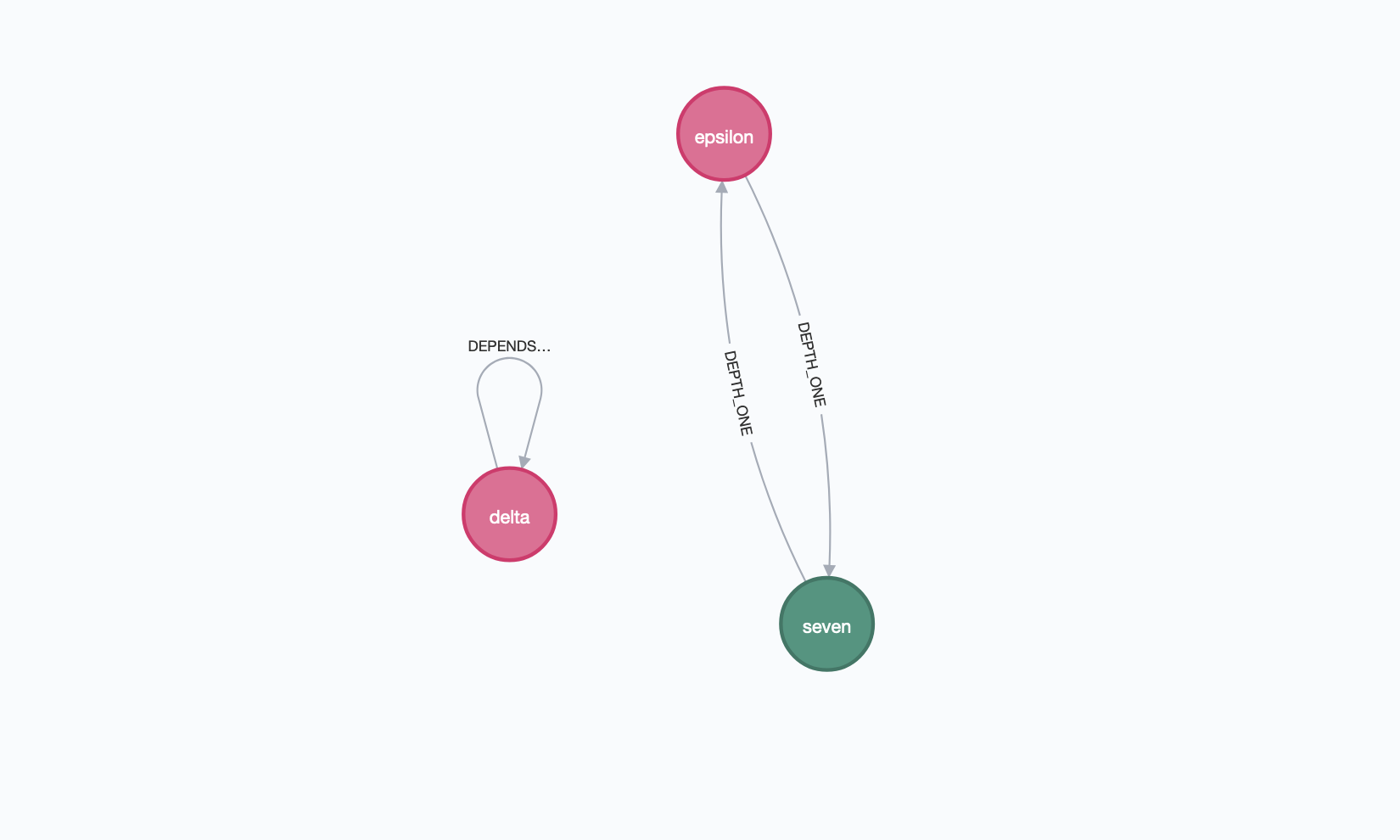Note that is allowed also a `maxDepth: 0`, returning only nodes with one or more self relationships:

``MATCH (m1:Start) WITH collect(m1) as nodes CALL apoc.nodes.cycles(nodes, {relTypes: ['DEPENDS_ON'], maxDepth: 0}) YIELD path RETURN path``